• 在大多数时候，我们的DBA都像是驾驶者一叶小舟行驶在一片平静的海面上，似乎一切都是那么和谐。不过平静总是暂时的，说不定什么时候海面会涌起巨浪，把这叶小舟拍碎在水面下的暗礁上。大海什么时候会...

在大多数时候，我们的DBA都像是驾驶者一叶小舟行驶在一片平静的海面上，似乎一切都是那么和谐。不过平静总是暂时的，说不定什么时候海面会涌起巨浪，把这叶小舟拍碎在水面下的暗礁上。大海什么时候会起波澜，这个我们很难预知，因此我们总是希望能够了解哪里有危险的礁石，好让我们的小舟远远的避开它。这实际上是大多数DBA在努力做的事情。问题是这片海太广了，而我们缺乏声纳、遥感设备，因此无法进行广泛的有效的探查。这实际上也是目前大多数DBA的困惑。
曾经和一个大企业的DBA交流，他们的信息系统数量爆炸式的增长，从以前他们DBA只需要管理好二三十个关键数据库开始才短短3、5年时间，他的团队管理的数据库实例已经高达400多个了，而运维人员还只有他一个正式编制人员和2个外包人员。按照运检规范，每天做一次巡检，都是不可能的事情。弄个监控软件，让运维人员有时间上去看两眼指标，也是不现实的事情。他们现在需要的是一套能够帮他们在后台默默值守的监控系统，没事时候都不需要打开监控去看，而真正有问题的时候，发个短信给他们，他们再上去处置就可以了。这个需求提的似乎并不高，不过要做到这一点十分不容易。传统的网管式的运维自动化系统，不论是开源的zabbix、prometheus还是商用的Precise I3，都无法提供这样的能力，让他在没有收到报警短信的时候可以相信系统没有可以令他手忙脚乱的隐患。这些系统要么就是每天发送数千条基线、日志告警，要么就是什么都不告诉他。
另外一个案例来自于某银行，他们的一个DBA管理着50多套B级系统的数据库，其中不少B级系统是银监会和银联监管的，一旦出现故障导致业务停运超过30分钟，大行长都要去银监会做解释。这些让他们提心吊胆的数据库系统让他们头痛的问题是，他们就像平静的海湾一样，似乎安全无害，而突如其来的某个风暴会让他们措手不及。他们每天都花时间认真去监控的时候，啥事都没有，而他们去忙一些其他事情的时候，突然就会闹点幺蛾子，让他们忙活半天。当我和他们说他们需要一个DB AIOPS系统，对这几十套数据库做一些深入的分析的时候，他们还在怀疑是不是有这个必要。他们每年都会请专家对这些系统做几次巡检，也没有发现过什么重大的隐患。当他们看到我们利用离线的运维监控数据生成的一份20多页的深度检查报告中的50多类关键隐患的时候，吓了一大跳。他们认为十分安全可靠的这些数据库中，居然有这么多的隐患，而且有不少都是高风险隐患。特别是他们看到某个系统中有一条SQL在半小时时间里执行了100万次，每次都返回0条数据的时候，更是吓出了一身冷汗，一旦这条业务逻辑存在问题的SQL的执行计划出现一点偏差，那么这套系统就完蛋了。
实际上DBA需要AIOPS来提升运维自动化系统中的分析能力，传统的靠人去看的监控系统已经越来越难给DBA提供有效的支撑了。而AIOPS以前关注的领域都在信息系统运行的宏观层面。通过AIOPS对信息系统的全链路进行分析，发现可能存在的异常，并且通过异常特征的建模，发现异常可能出现的位置，是网络、中间件、数据库还是存储系统。做出一个初步的定位就可以了。在这些运维实践中，AIOPS发挥了其特长，效果很好。不过在微观运维领域，比如在数据库运维领域，利用AI算法来发现数据库可能存在的潜在风险，并且定位风险可能的原因，指导运维人员消缺。这一点不仅仅需要高水平的数据处理算法，还需要十分强大的知识库。
昨天我在讨论异常衍生路径的时候，也提到了知识库的问题，知识库的积累需要大量的专家资源、大量的实际应用案例，是需要时间去沉淀的。一个AIOPS系统如果要深入到微观的领域去发挥作用，离开这些知识库是行不通的。除非一个企业能够聚集一批专家对AI算法发现的系统异常进行人工分析，否则这些专家的分析能力必须数字化。数字化监控还是相对容易的，我们把SNMP的MIBS库中的指标抄过来，就已经可以构建一个相对完善的监控系统了，再找一个开源的监控平台，让一些专家配一些基线，数据库监控平台就构建起了了。而如何把专家脑子里的那些干货数字化，是个更为复杂的问题。首先是专家愿意不愿意把自己赖以生存的看家本领数字化了。其次是如何数字化这些专家知识。事实上，后者还是有解的，前者就真的需要商榷了。构建一个开源的，开放的知识库项目有可能是解决这个问题的一个方法，我为人人，人人为我的大同世界是令人向往的，虽然实现起来还是困难重重。

展开全文运维 数据库 算法 大数据 java
• 想要实现一个纪念碑谷中的海面效果，最初的设想是在vertex中，修改顶点坐标来实现，而且也实现出来了，代码： v2f vert(a2v v) { v2f o; float4 offset; offset.xyzw = float4(0,0,0,0); float
想要实现一个纪念碑谷中的海面效果，最初的设想是在vertex中，修改顶点坐标来实现，而且也实现出来了，代码：

v2f vert(a2v v) {
v2f o;
float4 offset;
offset.xyzw = float4(0,0,0,0);

float sinx = sin(_Frequency * _Time.y + v.vertex.x * _InvWaveLength + v.vertex.y * _InvWaveLength + v.vertex.z * _InvWaveLength);
offset.y = sinx * _Magnitude;

o.pos = UnityObjectToClipPos(v.vertex+offset);

o.uv = TRANSFORM_TEX(v.texcoord,_MainTex);

o.norm = sinx;

o.worldPos =  mul(unity_ObjectToWorld,v.vertex).xyz;

o.sinx = sinx;

return o;
}

但是效果看上去总是怪怪的: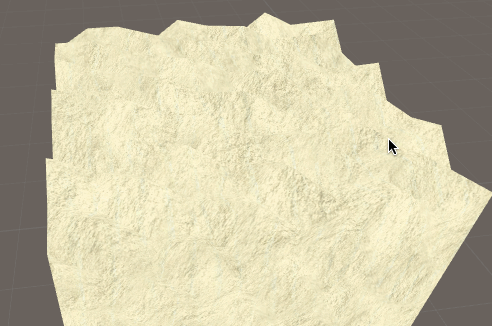想了老半天哪里怪，为啥跟想象中的不一样呢....我们看一下纪念碑谷中的效果:这差距也太大了吧...很明显，没有光效啊，那好，加上光效看看效果：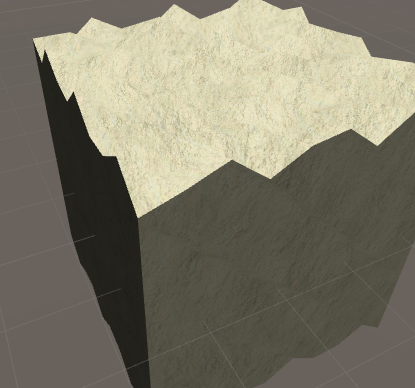结果还是一样，这是因为我们虽然处理的光，但是顶点的法线却并没有改变，不管顶点怎么变，亮度都是不会改变的，所以我们还需要计算一下法线，法线的计算我们要放在geometry中进行:

[maxvertexcount(3)]
void geom(triangle v2f IN, inout TriangleStream<v2f> triStream)
{
float3 v0 = IN.pos.xyz;
float3 v1 = IN.pos.xyz;
float3 v2 = IN.pos.xyz;

float3 vn = normalize(cross(v1 - v0, v2 - v0));

vn = UnityObjectToWorldNormal(vn);

v2f OUT;
OUT.pos = IN.pos;
OUT.worldNormal = vn;
OUT.worldPos = IN.worldPos;
OUT.uv = IN.uv;
triStream.Append(OUT);

OUT.pos = IN.pos;
OUT.worldNormal = vn;
OUT.worldPos = IN.worldPos;
OUT.uv = IN.uv;
triStream.Append(OUT);

OUT.pos = IN.pos;
OUT.worldNormal = vn;
OUT.worldPos = IN.worldPos;
OUT.uv = IN.uv;
triStream.Append(OUT);

}normalize(cross(v1 - v0, v2 - v0));这里对三角形的两条边做叉积，就得到了平面的法线。

看看现在的效果：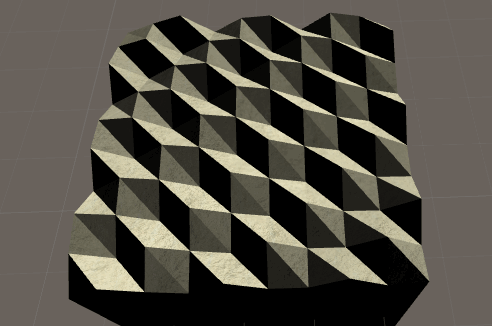这次有点接近了，但是没有变化，我们加点变化来看看,在vert中加上如下2句，对顶点的xz坐标也加上位移:

                offset.x =  sin(_Frequency * _Time.y + v.vertex.x);
offset.z =  sin(_Frequency * _Time.y  + v.vertex.z);再来看看: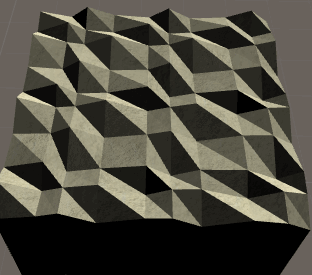总算是差不多了，想要达到更好的效果还需要进行一些细节的处理，有兴趣的朋友可以自己尝试.

Shader "Custom/Water" {
Properties {
_Color ("Color", Color) = (1,1,1,1)
_MainTex ("Main Tex", 2D) = "white" {}
_Magnitude ("Distortion Magnitude" , Float) = 1
_Frequency ("Distortion Frequency" , Float) = 1
_InvWaveLength("Distortion Inverse Wave Length",Float) = 10
_Speed("Speed",Float) = 0.5
}
Tags{"IgnoreProjector"="True"  "Queue"="Transparent" "RenderType"="Transparent"}

Pass{
CGPROGRAM

#pragma vertex vert
#pragma geometry geom
#pragma fragment frag

#include "Lighting.cginc"

sampler2D _MainTex;
float4 _MainTex_ST;
fixed4 _Color;
float _Magnitude;
float _Frequency;
float _InvWaveLength;
float _Speed;

struct a2v{
float4 vertex : POSITION;
float3 normal : NORMAL;
float4 texcoord : TEXCOORD0;
};

struct v2f {
float4 pos : SV_POSITION;
float3 worldNormal : TEXCOORD0;
float3 worldPos : TEXCOORD1;
float2 uv : TEXCOORD2;
};

v2f vert(a2v v) {
v2f o;
float4 offset;
offset.xyzw = float4(0,0,0,0);

float sinx = sin(_Frequency * _Time.y + v.vertex.x * _InvWaveLength + v.vertex.y * _InvWaveLength + v.vertex.z * _InvWaveLength);
offset.y = sinx * _Magnitude;
offset.x =  sin(_Frequency * _Time.y + v.vertex.x);
offset.z =  sin(_Frequency * _Time.y  + v.vertex.z);

o.pos = UnityObjectToClipPos(v.vertex+offset);
o.uv = TRANSFORM_TEX(v.texcoord,_MainTex);
o.worldPos =  mul(unity_ObjectToWorld,v.vertex).xyz;

return o;
}

[maxvertexcount(3)]
void geom(triangle v2f IN, inout TriangleStream<v2f> triStream)
{
float3 v0 = IN.pos.xyz;
float3 v1 = IN.pos.xyz;
float3 v2 = IN.pos.xyz;

float3 vn = normalize(cross(v1 - v0, v2 - v0));

vn = UnityObjectToWorldNormal(vn);

v2f OUT;
OUT.pos = IN.pos;
OUT.worldNormal = vn;
OUT.worldPos = IN.worldPos;
OUT.uv = IN.uv;
triStream.Append(OUT);

OUT.pos = IN.pos;
OUT.worldNormal = vn;
OUT.worldPos = IN.worldPos;
OUT.uv = IN.uv;
triStream.Append(OUT);

OUT.pos = IN.pos;
OUT.worldNormal = vn;
OUT.worldPos = IN.worldPos;
OUT.uv = IN.uv;
triStream.Append(OUT);

}
fixed4 frag(v2f i) : SV_Target {
//获取法线方向
fixed3 worldNormal = normalize(i.worldNormal);
//灯光方向
fixed3 worldLightDir = normalize(UnityWorldSpaceLightDir(i.worldPos));

fixed4 c = tex2D(_MainTex,i.uv) * dot(worldNormal,worldLightDir);

return c;
}
ENDCG
}
}
FallBack "Transparent/VertexLit"
}


展开全文unity shader
• 平面扩大成为海面，此时一定注意波长，小于最短波长会出现法线不对的情况。 （但有趣的是，失真有时会带来比较复杂的波面，参考 之前博文 的失真情况。如果不需要用到法线，还是很用的） 结果： 我还将...
目的：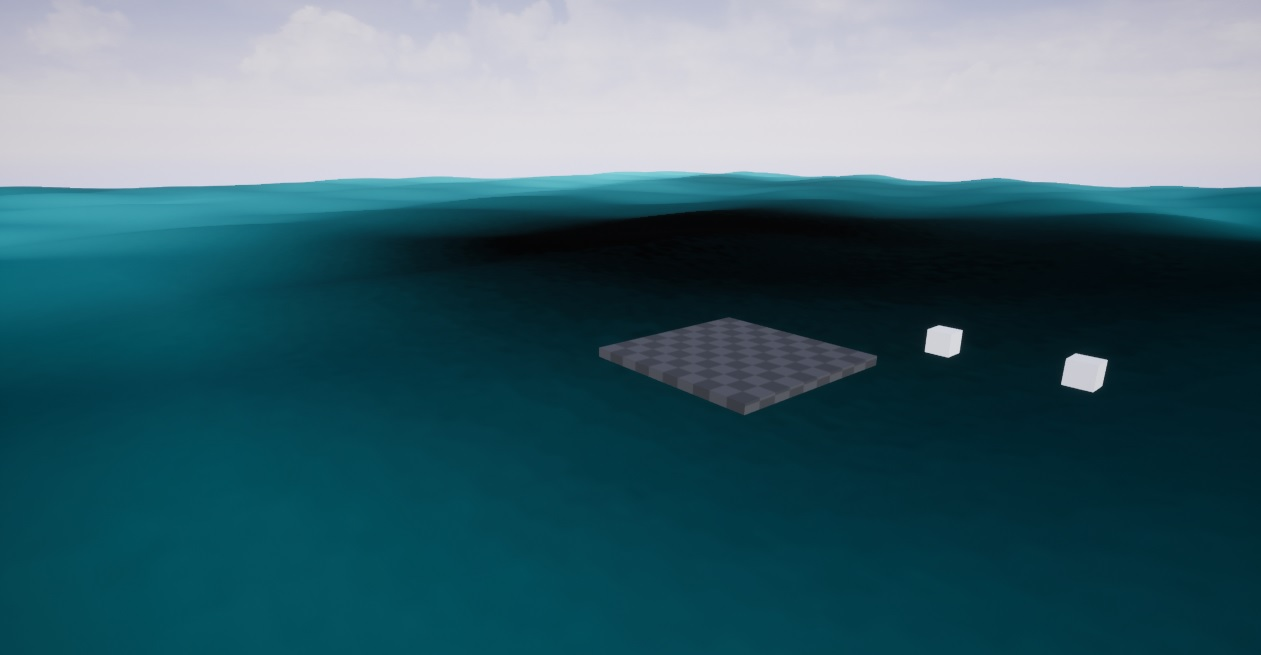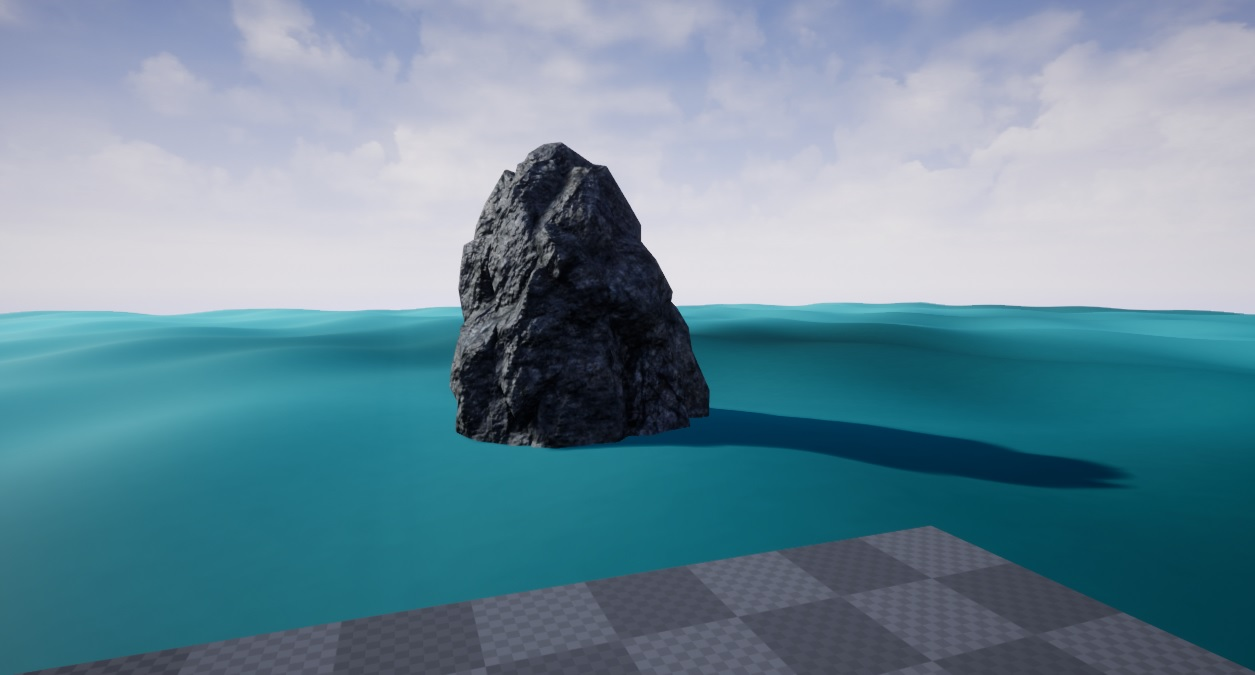参考：
《GPU Gems》
引言：
思路：
1.只能模拟一个方向简单的水波状态，波间距一致，缺少自然感。
2.波形状一致，单一，且与某些常见的海浪形状不一致。
下面，针对这两点分别改进。
1.通过叠加增加复杂感
没错，这个问题的解决方案很简单，我们当前不就只有一个方向的波么？多加两个，调调参数就完事了。
记得高中、大学数学学过的导数法则：

所以，添加多个波之后的方程也极其简单。
波面方程：

法线方程：

PS：
如果你知道傅里叶变换(Fourier transform)，那么就能意识到只要多加几个波，什么样复杂的水面都可以模拟出来。
因为几乎任何函数都能被拆解成傅里叶级数(Fourier series)，包括不连续的函数。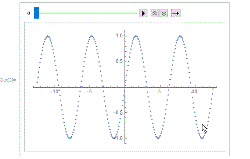级数越多，“分辨率”越高。这个特性是不是像极了我们之前提到过的泰勒公式(Taylor's formula)？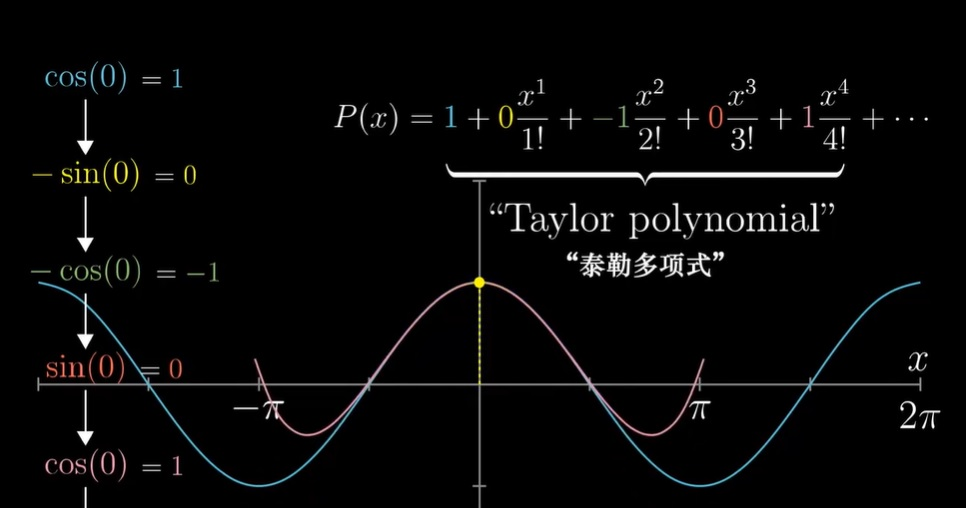在复分析(Complex Analysis)中，它们两是"两位一体"。（参考《复分析可视化方法》）
2.添加更有浪尖的Gerstner波
2.1Gerstner波方程
根据前文所说，我们的Sin函数不太像某些真实的浪，往往拥有锋利的浪尖。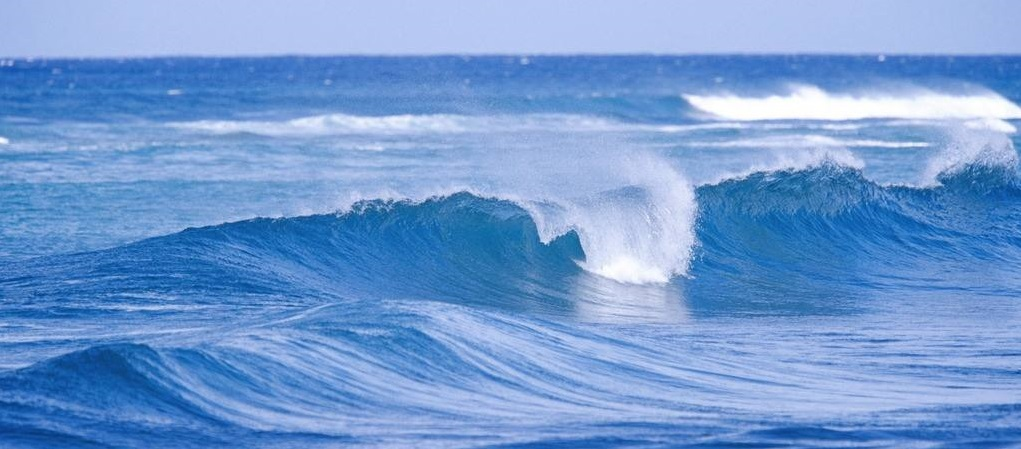图形学前辈们在我们目前的函数上进行改进，发明了Gerstner波。
形状如下：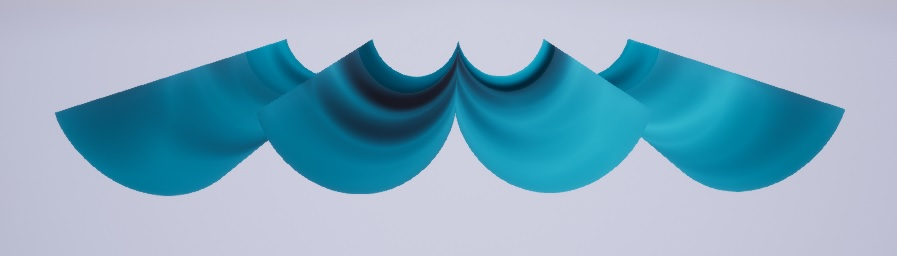公式（显然这是多个Gerstner波的叠加）：

别忘了其中，只是为了简化写法。
注意：我在此对《GPU Gem》上的Gerstner波公式改了写法。一是为了衔接我们之前推导的公式，改变了写法。
二是原文的参数冗余，不利于理解。但确为完全一致的公式。
可以看出，Z方向上的函数与我们的函数一致，只是x,y方向加了一些东西。
在此我设，理解Gerstner波就在于理解。
下面是我的理解。
我们要干什么？我们要把波峰搞尖。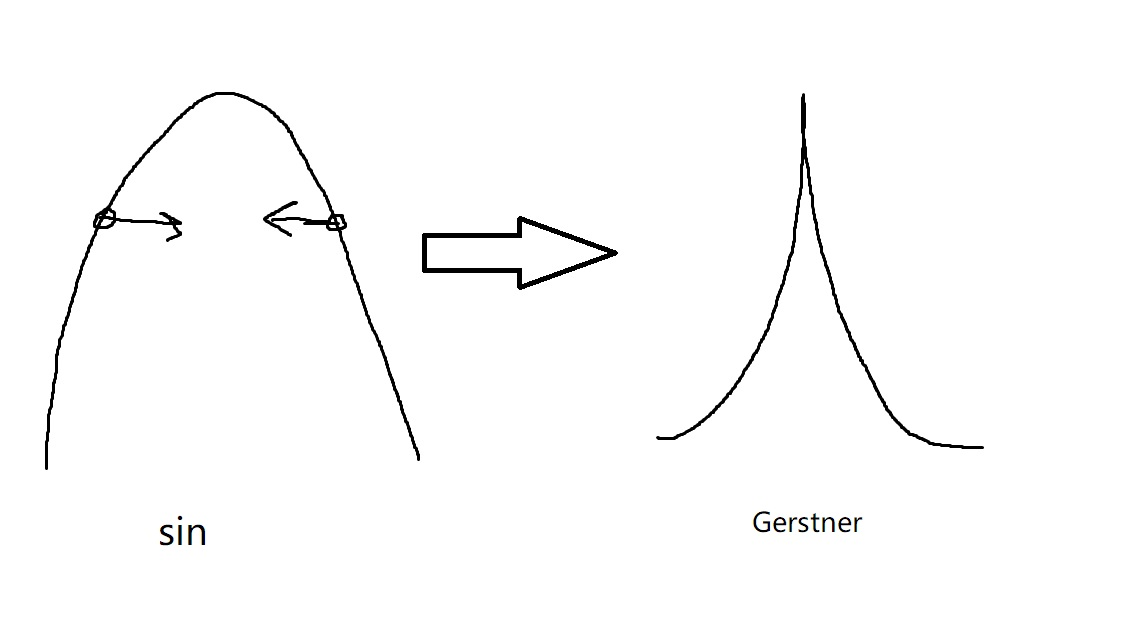看到没有？如果我把每个点往法线相反方向挤一挤，这个峰就尖了。
x轴的往法线x分量负方向挤一挤，y轴的往法线y分量负方向挤一挤。
当这个挤的量为法线在x/y轴的分量，会导致法线朝上的被挤的少，法线水平得挤的多，就成了尖峰的样子。
再加两个参数控制一下挤的程度，就完事了。
这也就是为什么L(x),L(y)会这么像我们原来的法线

那为啥前面的系数是这样呢？原来前辈早替不懂原理的美术们想好了，这样设计，Q只要保证在[0,1]就行。取0时就和我们原来一样，取1时浪尖最尖。Q大于1浪尖就不对了，浪尖出现环状：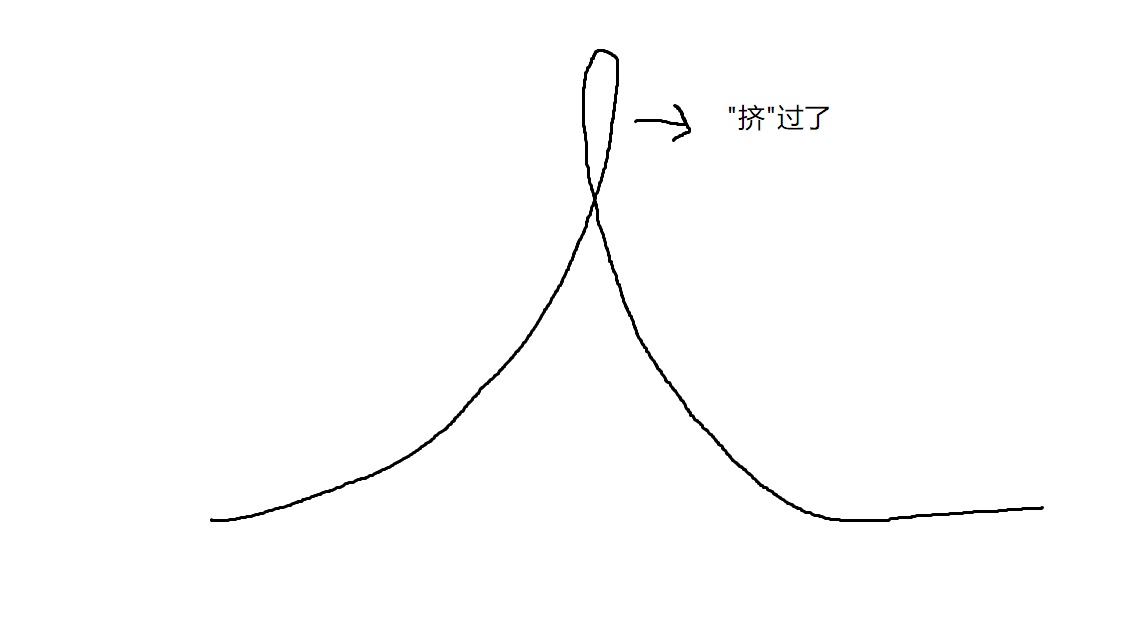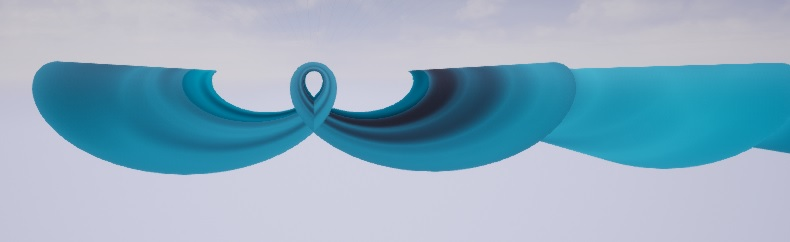为啥Q大于1就会这样呢？
《GPU Gems》只简单提了一下在频域内可发现并解决这个问题。关于频域我准备在以后必要的时候再回过头来讨论。
我现在给出一个简单的几何证明。波函数被简化。
挤之前的点，
挤之后变成了点
直观上来说，对于Sin函数，仅看处于的点x，x+L(x)还应该<=T/4。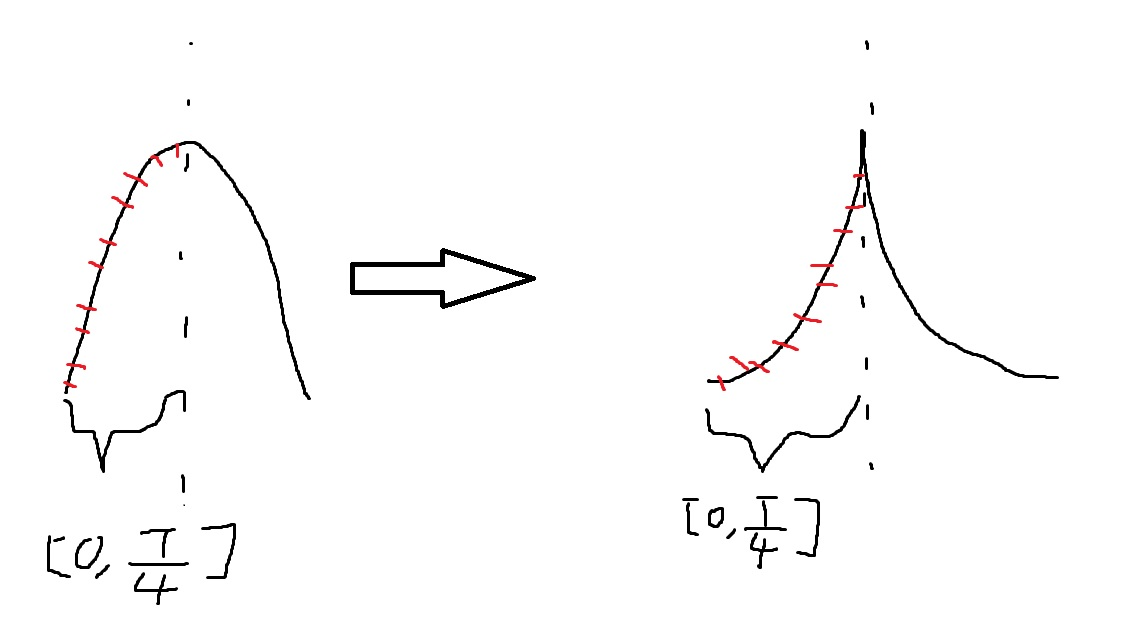有不等式

设u=wx，化简得：

接下来得过程很像高三数学大题（江苏）。
不论是画出图形，还是研究单调性。你会发现函数单调减。
照理来说，证明当u=pi/2时，G(u)=1，就行了。但cos(pi/2)=0,G(u)不存在。
只能求极限，u->pi/2,求lim G(u)。
直接上高数洛必达法则(L'Hopital's rule)

两个趣点：
1.没错，咱江苏数学就是有高数内容，咱就喜欢超纲，傻了8。可惜以后都是全国卷了...
2.别忘了洛必达法则是土豪洛必达买来的，没错，有钱就是可以为所欲为。
2.2Gerstner波的法线方程
记得之前博文关于法线推导的推导一吗？我本人不赞成这种方法。但结果这儿发现了它的好处。
对于不存在z=Z（x,y）形式，而且全方程又很难列出来，但知道3分量各自解析式的情况，推导一就可用。
不再细述过程，结果：

这样关于实现Gerstner波的内容就完整了。
步骤：
1.对材质进行修改。
运用上我们的公式，将结果给予WPOffset,Normal。
//wave normal
float3 re;
float pi=3.14f;

float w=2*pi/T;
float k=dot(float2(OriPos.x,OriPos.y),dir);
float phase=2*pi*frac(time/speedCycle)*phaseDir;
//wave2
float w2=2*pi/T2;
float k2=dot(float2(OriPos.x,OriPos.y),dir2);
float phase2=2*pi*frac(time/speedCycle2)*phaseDir2;
//wave3
float w3=2*pi/T3;
float k3=dot(float2(OriPos.x,OriPos.y),dir3);
float phase3=2*pi*frac(time/speedCycle3)*phaseDir3;
re.x=-A*cos(w*k+phase)*w*dir.x-A2*cos(w2*k2+phase2)*w2*dir2.x-A3*cos(w3*k3+phase3)*w3*dir3.x;
re.y=-A*cos(w*k+phase)*w*dir.y-A2*cos(w2*k2+phase2)*w2*dir2.y-A3*cos(w3*k3+phase3)*w3*dir3.y;
re.z=1;
//wave4
float3 wave4N;
float w4=2*pi/T4;
float k4=dot(float2(OriPos.x,OriPos.y),dir4);
float phase4=2*pi*frac(time/speedCycle4)*phaseDir4;
float S=sin(w4*k4+phase4);
float C=cos(w4*k4+phase4);
wave4N.x=-dir4.x*w4*A4*C;
wave4N.y=-dir4.y*w4*A4*C;
wave4N.z=1-Q*w4*A4*S;
re+=wave4N;
return re;
//WPOffset
float3 re;
float pi=3.14f;
re.x=0.0f;
re.y=0.0f;
float phase=2*pi*frac(time/speedCycle)*phaseDir;
float phase2=2*pi*frac(time/speedCycle2)*phaseDir2;
float phase3=2*pi*frac(time/speedCycle3)*phaseDir3;
float wave1=A*sin(2*pi/T*dot(float2(OriPos.x,OriPos.y),dir)+phase);
float wave2=A2*sin(2*pi/T2*dot(float2(OriPos.x,OriPos.y),dir2)+phase2);
float wave3=A3*sin(2*pi/T3*dot(float2(OriPos.x,OriPos.y),dir3)+phase3);
re.z=wave1+wave2+wave3;
//
//wave4,Gerstner
float3 wave4;
float w4=2*pi/T4;
float k4=dot(float2(OriPos.x,OriPos.y),dir4);
float phase4=2*pi*frac(time/speedCycle4)*phaseDir4;
wave4.x=Q*1/w4*dir4.x*cos(w4*k4+phase4);
wave4.y=Q*1/w4*dir4.y*cos(w4*k4+phase4);
wave4.z=A4*sin(w4*k4+phase4);
re+=wave4;
return re;
2.将平面扩大100倍，调整参数
平面扩大成为海面，此时一定注意波长，小于最短波长会出现法线不对的情况。
（但有趣的是，失真有时会带来比较复杂的波面，参考之前博文的失真情况。如果不需要用到法线，还是很用的）
结果：
我还将BaseColor简单乘上一个贴图，显得有趣些。
3Sin不加Gerstner: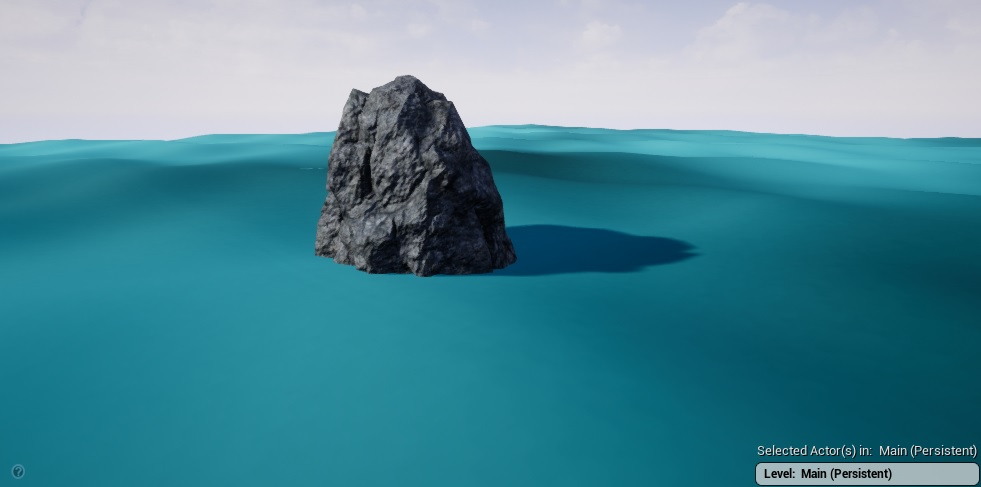仔细看会发现还是有重复的模式(Pattern)出现，在高处俯视更加明显，毕竟只有3个sin波。但是你去看看一些游戏中的海面，说不定更加明显。而且我们对其表面材质和光照还未关注，只有造型，游戏中的海面通过其他东西增加了你的真实感。
增加Gerstner，模拟一阵一阵冲岸的小尖浪，或者灾难片中的百尺巨浪，之后要解决固定波长的问题。
因为这种浪速度较快要持续出现，固定波长的话就显得十分人工。
这条斜面大，浪脊分明的波就是Gerstner波：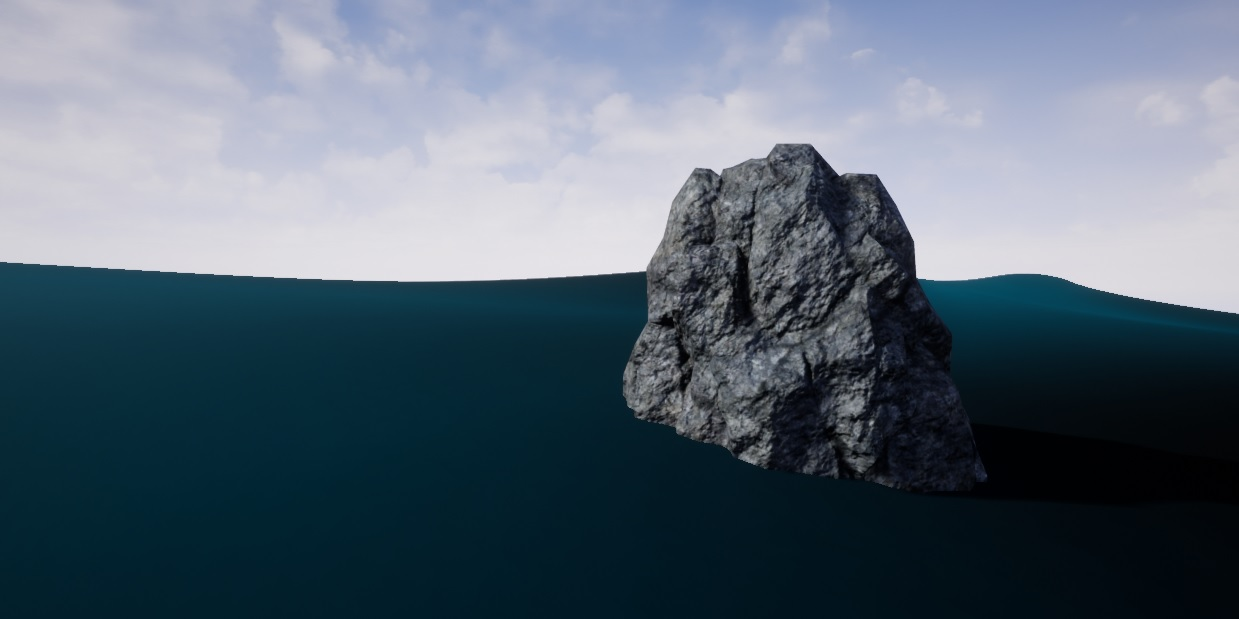结语：
下一篇会解决Gerstner波，波长固定的问题，并开始研究水波模拟的表面材质，光照部分。


展开全文• 太菜啦，这都不会。...二分距离，检查是否存在一个距离这么远的点，具体做法就是将所有半平面对应的直线往左边移动这么远，然后看看交是否为空即可。 代码 #include using namespace std; const int m
太菜啦，这都不会。。。
点到海的距离是点到海的最小距离，然后想让这个值最大。想到了啥？不就是应该用二分吗？太菜了。

把这个n个点的凸多边形的岛看做n个半平面的交。
二分距离，检查是否存在一个距离海这么远的点，具体做法就是将所有半平面对应的直线往左边移动这么远，然后看看交是否为空即可。

代码

#include<bits/stdc++.h>
using namespace std;
const int maxn = 110;

struct Point
{
double x,y;
Point(double x=0,double y=0):x(x),y(y){}
};

typedef Point Vector;

const double eps = 1e-10;
int dcmp(double x)
{
if(fabs(x)<eps) return 0;
else return x<0?-1:1;
}

Point operator + (Point p,Vector v)
{
return Point(p.x+v.x,p.y+v.y);
}

Vector operator - (Point A,Point B)
{
return Vector(A.x-B.x,A.y-B.y);
}

Vector operator * (Vector V,double t)
{
return Vector(V.x*t,V.y*t);
}

Vector operator / (Vector V,double t)
{
return Vector(V.x/t,V.y/t);
}

double Dot(Vector A,Vector B)
{
return A.x*B.x+A.y*B.y;
}

double Cross(Vector A,Vector B)
{
return A.x*B.y-A.y*B.x;
}

double Len(Vector V)
{
return sqrt(Dot(V,V));
}

Vector Normal(Vector V)
{
double L=Len(V);
return Vector(-V.y/L,V.x/L);
}

double angle(Vector v)
{
return atan2(v.y,v.x);
}

struct Line
{
Point p;
Vector v;
double ang;
Line(Point p=Point(),Vector v=Vector()):p(p),v(v)
{
ang=angle(v);
}
bool operator < (const Line& rhs) const
{
return ang<rhs.ang;
}
};

bool OnLeft(Line L,Point P)
{
return Cross(L.v,P-L.p)>0;
}

Point GetLineInterSection(Line L1,Line L2)
{
Vector u=L2.p-L1.p;
double t=Cross(u,L2.v)/Cross(L1.v,L2.v);
return L1.p+L1.v*t;
}

int GetHalfPlaneInterSection(Line* L,int n,Point* Poly)
{
Point* p=new Point[n];
Line* q=new Line[n];
int first,last;
q[first=last=0]=L;
for(int i=1;i<n;i++)
{
while(last>first&&!OnLeft(L[i],p[last-1])) last--;
while(last>first&&!OnLeft(L[i],p[first])) first++;
q[++last]=L[i];
if(dcmp(Cross(q[last].v,q[last-1].v))==0)
{
--last;
if(OnLeft(q[last],L[i].p)) q[last]=L[i];
}
if(first<last) p[last-1]=GetLineInterSection(q[last],q[last-1]);
}
while(first<last&&!OnLeft(q[first],p[last-1])) last--;
if(last-first<=1) return 0;
p[last]=GetLineInterSection(q[last],q[first]);
int m=0;
for(int i=first;i<=last;i++) Poly[m++]=p[i];
return m;
}

{
double x,y;
scanf("%lf %lf",&x,&y);
return Point(x,y);
}

int n;
Point P[maxn];
Point Poly[maxn];
Line L[maxn];
Line LL[maxn];

const double EPS=1e-7;

int main()
{
while(scanf("%d",&n)==1&&n)
{
for(int i=0;i<n;i++)
for(int i=0;i<n;i++)
L[i]=Line(P[i],P[(i+1)%n]-P[i]);
double l=0;
double r=3e8;
while(r-l>EPS)
{
double m=l+(r-l)/2;
for(int i=0;i<n;i++)
{
LL[i]=L[i];
LL[i].p=LL[i].p+Normal(LL[i].v)*m;
}
if(!GetHalfPlaneInterSection(LL,n,Poly)) r=m;
else l=m;
}
printf("%.6lf\n",l);
}
return 0;
}



展开全文• ”我想了想回他一句：“800年以后，估计没问题。”   一个同事大声问我：“炒股了吗？”我说，“没有。我没什么精力。”“靠，要什么精力啊。现在不炒股，就是傻×”。   真心祝它接着涨下去，因为已经有这么多...互联网
• 小建议 建议投，走内推，内推渠道获取途径可以看我另外一篇专门讲解内推，春招，秋招，大学。 宇宙条是春招第一家给我面试机会的公司，收到面试邀约的时候没抱太大希望，全世界都知道宇宙条唯算法论，算法这块是...算法
• 今天给大家分享一下这组的色调怎么调以及我平常修皮肤的步骤，大家慢慢看。 我们来看下原图，原图略灰，...第一步：LR调色基本：将色温往左边降，整体感觉会变清爽的色调，蓝色的天空和的颜色就会稍微突出一些。...
• 求职类网站投递简历（不是太推荐，适合投）； 除了这些方法，我也遇到过这样的经历：有些大公司的一些部门可能暂时没招够 人，然后如果你的亲戚或者朋友刚好在这个公司，而你正好又在寻求 offer，那 么面试机会...网络互联 程序员 移动开发 Android
• 还有就是觉得轻松。后来看了诗，去顺着想一想，我都想在海边种块花地，在花成长时看着。再后来，和C聊天时才知道，这不是轻松而是挣扎，甚至和死都有关系。简短的几句c
• 而且我发现，现在面试 Java 岗位，无论什么规模的公司，一般面试官着就会问到 JVM 相关的问题，什么线程、内存模型、JVM 运行时内存、垃圾回收与算法、GC 垃圾收集器、JAVA IO/NIO 、JVM 类加载机制等等知识点...
• 6. 广告系统架构：要自行车！ 一句话解释广告系统架构：“道生一，一生二，二生三，三生万物。” 场景1 时间：13 :40 地点：北邮北门 人物：快车司机，我 事件：去面试 状态：忐忑 坐在车里...计算广告 机器学习 推荐系统
• 如相识，九月茶花满路开』。 六、和萧峰的对谈，引李白之诗篇，表明对和平的渴望：『烽火燃不息，征战无已时。 野战格斗死，败马号鸣向天悲。鸟鸢啄人肠，冲飞上挂枯树枝。士卒涂草莽，将军空尔 为。乃知兵者是...语言
• 中层，瞄准86年-91年，团队主管最好是87年、88年的”。 “中层管理者年轻化，条件差不多，选年轻的”。 工作越来越像职业运动员，28岁到达巅峰，35岁必须退役。你以为你做的是脑力劳动，其实你就是个做体力劳动...
• 跟我一起工作过的同事里，说起来好玩的爱好就包括：天天在车库里打造奇形怪状的自行车，休一个无薪假期去帮别人竞选总统，每个周末都 跑到一个从没去过的地方留下到此一游照，研究古音韵学，出远调查海洋生物，...
• 6. 广告系统架构：要自行车！ 一句话解释广告系统架构：“道生一，一生二，二生三，三生万物。” 场景1 时间：13 :40 地点：北邮北门 人物：快车司机，我 事件：去面试 状态：忐忑 坐在车里...计算广告 机器学习 广告系统
•  生活上没太大变化，从合租一个卧室到独租一间，一个厅隔贴满了粉红色的墙纸，一套粉红色的四件套，适逢阳光明媚从阳台照进来的阳光，即便是租住的厅隔，那一刻真的也算满足了。其他的一共去了三个地方旅游，一次...
• 高铁与动车的区别到底在哪里？磁悬浮列车又是什么鬼？...车轮滚过钢轨连接处时，车轮震动、车厢震动、地面也震动，列车行驶一点儿都不平稳，简直就像在大风大浪的海面上行船一样，不能平稳前进，速度就不能提高，否
• 前言 ...了一个月其他公司后，我找了我实验室的学长内推我，后面就有了这5次面试。学长还把他认为很有价值的刷面试的文档、视频以及学习路线分享给了我（文末有分享） 下面简单的说一下我的...java 编程语言 人工智能
• 一面： 1.自我介绍。 2.讲自己最熟悉的项目，测试流程说一下，测试的产品介绍一下。讲了自己实习时候做的测试工作。 3.写个代码，很简单，字符串...我的HR只有一个，不知道有的两个有的一个是意思。 ...查看全文
• 文章目录1. 习惯性唠叨点2. 什么造就了爬虫3. 为什么要反爬虫4. 反反爬虫的权重5. 高级的反爬手段6. 爬虫的发展方向7. ...
• 它把煎饼人在一定程度上提升了下，对某一块了解的很深，但是又不缺乏知识的广度。我很赞成这个说法，如果当时也能意识到这一点，那该多好。有的人也说过： 种一棵树的最好时机是十年前，其次是现在。 但从某种意义...
• 人太懒了，就随便说一下       先说下我们计算机相关专业公司招招聘的时的岗位，大方向有两种：研发岗和算法岗。具体细分就很多了。 一般在一个部门里两种岗位都有，人们是相互协作的...
• 这是我17年的经历，觉得还挺励志的，希望能帮助到一些正在迷茫的同学。前言是去美团送外卖了？有配电瓶车吗？答：亲，有配的哦，开起来贼顺滑。啊呸，说了，咱进的是正儿八经的技术部门。正文咸鱼...
• 秉承着追求科（wu）学（mei）严（jia）谨（lian）的目标，越来越多的科学家想要在有限的经费内获得更多覆盖更全的序列信息，于是Apprill等科学家在515F改善的基础上，进一步改善了806R端（嗯，也是只改了一个兼并...
• 文章目录一、借助翻译学英语二、沉迷翻译，自得其乐三、译泛舟，浪花朵朵（一）科普文章的翻译（二）散文的翻译（三）诗词的翻译1、英诗汉译2、汉诗英译四、译诗是灵魂的亲密接触 译泛轻舟 浩瀚译泛轻舟， 只...
• ## 【字节面试题】ZJ27 字典树是啥

千次阅读 多人点赞 2020-07-17 23:44:13
想要看更加舒服的排版、更加准时的推送 ...if steps *= 10 print(cur) 推荐阅读： Pandas对海平面温度异常进行分析 机器学习中必须要会用的模块 这些基础题你能答上来几个？ 还不知道怎么运行Python代码，快来撸代码数据结构 算法 python 字符串
• 我们假设跑道相对于海平面的高度是0英尺，然后沿跑道方向向后移动3海里到最终进近定位点。1海里大概等于6076英尺（1.852千米），3海里就是18228英尺，我们以此为例，使用三角函数中的正切函数来计算飞机正确的高度。......